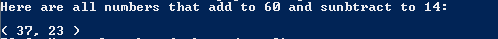# Assignment #115

## Code

```/// Name: Jonathan Stine
/// Period: 5
/// Program Name: Number Puzzle I
/// File Name: NumberPuzzle.java
/// Date Finished: 1/27/2016

public class NumberPuzzle {

public static void main( String[] args ) {

System.out.println( "Here are all numbers that add to 60 and sunbtract to 14:" );
System.out.println();

for( int i=0; i<=60; i++ ) {

for( int n=0; n<=60; n++ ) {
int c = n+i;
int d = n-i;

if ( c == 60 ) {
if ( d == 14 ) {
System.out.println( "( " + n + ", " + i + " )" );
}
}
}
}

}
}

```

### Code Output